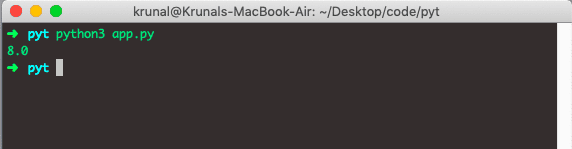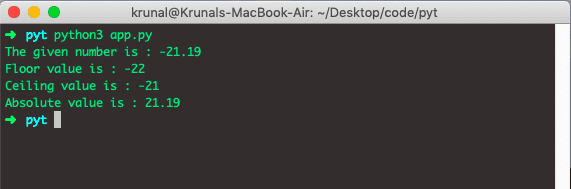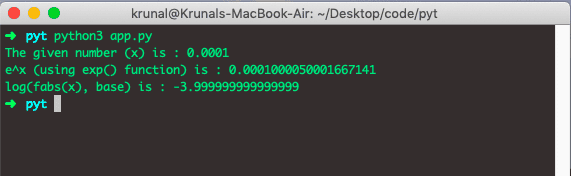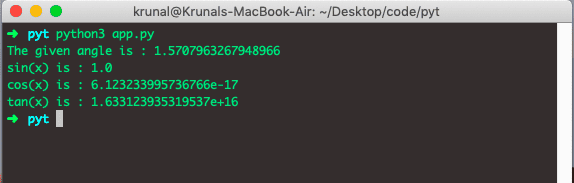# Python math: The Complete Guide

To use the math in Python, import the module using import math statements in your program. After that, you can call any method of that module. In Python, some mathematical operations can be performed easily in the math module.

## Most Used Python Math Functions

1. fabs(): It returns the absolute value of x.
2. ceil():- It returns the smallest integer value greater than or equal to x.
3. floor():- It returns the largest integer less than or equal to x.
4. factorial():- It returns the factorial of x.
5. gcd():- This function is used to compute the greatest common divisor of 2 numbers mentioned in its arguments. This function works in python 3.5 and above.

## Python math

Python math is a built-in standard module used to work with complex scientific calculations. Mathematical functions like evaluating complex mathematical operations, such as trigonometric operations, logarithmic operations, etc., are under the math module. However, the math module does not support complex data types. For that, you can use the cmath module as the complex counterpart.

Let’s take some examples of the Math module.

```# app.py

import math

data = 21.6
print('The floor of 21.6 is:', math.floor(data))```

See the output.The floor() function returns the highest integer value smaller than the number. If the number is already the integer, then the same number is returned.

Let’s see the value of PI.

```# app.py

import math

print('The value of PI:',math.pi)```

Okay, let’s see the output below.## math.pow

The math.pow() is a built-in Python library function that returns the value of x to the power of y (x²). To calculate the power in Python, use the math.pow() function. Python offers to compute the power of a number and hence can make calculating the power easier.

### Syntax

See the following syntax of Power.

`pow(x,y,z)`

### Arguments

The pow() method returns the value of x to the power of y (xy).

 x A number, the base y A number, the exponent z Optional. A number, the modulus

### Example

```# app.py

import math

print(math.pow(2, 3))
```

The output is the following.## Python ceil() function

The ceil() function returns the smallest integer value greater than the number. If the number is already an integer, then the same number is returned.

The method ceil() returns the ceiling value of x – the smallest integer, which is not less than x.

```# app.py

import math

data = 21.6
print(math.ceil(21.6))```

See the output below.Let’s see more examples.

```# app.py

import math

number = -21.19

print('The given number is :', number)
print('Floor value is :', math.floor(number))
print('Ceiling value is :', math.ceil(number))
print('Absolute value is :', math.fabs(number))```

The output is the following.## math.exp

The math.exp() is a built-in Python library method that returns E raised to the power of x (Ex). The “E” is the base of the natural system of logarithms (approximately 2.718282) and x is the number passed to it. The exp() method is used to get e^x

## math.log

The math.log() is a built-in Python method that returns the natural logarithm of a number, or the logarithm of a number to base. Let’s see an example of the Python Math exp() and log() functions.

```# app.py

import math

number = 1e-4
print('The given number (x) is :', number)
print('e^x (using exp() function) is :', math.exp(number)-1)
print('log(fabs(x), base) is :', math.log(math.fabs(number), 10))```

See the output below.## Python math trigonometric functions

All the trigonometric functions are available in python math module, so you can easily calculate them using sin()cos()tan()acos()asin()atan() etc functions.

Also, you can convert angles from degree to radian and radian to degree. See the example code.

```# app.py

import math

angleInDegree = 90

print('The given angle is :', angleInRadian)

See the output below.## All the Python Math Functions Table

See the below table, which has every Python Math module’s function with a description.

Function Description
ceil(x) Returns the smallest integer value greater than or equal to x.
copysign(x, y) Returns x with a sign of y
fabs(x) Returns the absolute value of x
factorial(x) Returns the factorial of x
floor(x) Returns the largest integer less than or equal to x
fmod(x, y) Returns a remainder when x is divided by y
frexp(x) Returns a mantissa and exponent of x as the pair (m, e)
fsum(iterable) Returns the accurate floating-point sum of values in the iterable
isfinite(x) Returns True if x is neither infinity nor a NaN (Not a Number)
isinf(x) Returns True if x is the positive or negative infinity
isnan(x) Returns True if x is the NaN
ldexp(x, i) Returns x * (2**i)
modf(x) Returns a fractional and integer parts of x
trunc(x) Returns a truncated integer value of x
exp(x) Returns e**x
expm1(x) Returns e**x – 1
log(x[, base]) Returns the logarithm of x to the base (defaults to e)
log1p(x) Returns the natural logarithm of 1+x
log2(x) Returns the base-2 logarithm of x
log10(x) Returns the base-10 logarithm of x
pow(x, y) Returns x raised to the power y
sqrt(x) Returns the square root of x
acos(x) Returns the arc cosine of x
asin(x) Returns the arc sine of x
atan(x) Returns the arc tangent of x
atan2(y, x) Returns atan(y / x)
cos(x) Returns the cosine of x
hypot(x, y) Returns the Euclidean norm, sqrt(x*x + y*y)
sin(x) Returns the sine of x
tan(x) Returns the tangent of x
degrees(x) Converts angle x from radians to degrees
acosh(x) Returns the inverse hyperbolic cosine of x
asinh(x) Returns the inverse hyperbolic sine of x
atanh(x) Returns the inverse hyperbolic tangent of x
cosh(x) Returns the hyperbolic cosine of x
sinh(x) Returns the hyperbolic cosine of x
tanh(x) Returns the hyperbolic tangent of x
erf(x) Returns the error function at x
erfc(x) Returns the complementary error function at x
gamma(x) Returns the Gamma function at x
lgamma(x) Returns the natural logarithm of the absolute value of the Gamma function at x
pi Mathematical constant, the ratio of the circumference of a circle to its diameter (3.14159…)
e mathematical constant e (2.71828…)

That’s it for this tutorial.

## Recommended Posts

Python sqrt()

Python abs()

Python eval()

Python complex()

Python pow()

This site uses Akismet to reduce spam. Learn how your comment data is processed.